﻿ Smooth Bootstrap Methods on External Sector Statistics

### Smooth Bootstrap Methods on External Sector Statistics

Acha Chigozie K, Acha Ikechukwu AOPEN ACCESSPEER-REVIEWED

## Smooth Bootstrap Methods on External Sector Statistics

Acha Chigozie K1,, Acha Ikechukwu A2

1Department of Statistics, Michael Okpara University of Agriculture Umudike, Abia State, Nigeria

2Department of Banking and Finance, University of Uyo, Uyo, AkwaIbom State, Nigeria

### Abstract

The investigation of the possibility of a significant difference existing in the parametric and nonparametric bootstrap methods on external sector statistics, and establishing the sample data distribution using the smooth bootstrap is the focus of this study. The root mean square error (RMSE) and the kernel density will be used on the test statistic θ in the determination of such difference. Establishing this difference will lead to more detailed study to discover reasons for such difference. This will also aid the Nigeria economy to aim at improving the performance of the external sector statistics (ESS). The study used secondary data from Central bank of Nigeria (1983-2012). Analysis was carried out using R-statistical package. In the course of the analysis, 17280 scenarios were replicated 200 times. The result shows a significant difference between the performances of the parametric and nonparametric smooth bootstrap methods, namely; wild and pairwise bootstrap respectively. The significantly better performance of the wild bootstrap indicate the possible use of this technique in assessment of comparative performance of ESS with a view to further understanding the better performers in order to identify factors contributing to such better performance. Also, when the sample size and the bootstrap level are very high, the smooth bootstrap or kernel density estimates outperform the pair wise bootstrap notwithstanding that they are nonparametric methods. The kernel density plots revealed that the sampling distribution of the ESS was found to be a Chi-square distribution and was confirmed by the smooth bootstrap methods.

### At a glance: Figures

12
Prev Next

• K, Acha Chigozie, and Acha Ikechukwu A. "Smooth Bootstrap Methods on External Sector Statistics." International Journal of Econometrics and Financial Management 3.3 (2015): 115-120.
• K, A. C. , & A, A. I. (2015). Smooth Bootstrap Methods on External Sector Statistics. International Journal of Econometrics and Financial Management, 3(3), 115-120.
• K, Acha Chigozie, and Acha Ikechukwu A. "Smooth Bootstrap Methods on External Sector Statistics." International Journal of Econometrics and Financial Management 3, no. 3 (2015): 115-120.

 Import into BibTeX Import into EndNote Import into RefMan Import into RefWorks

### 1. Introduction

The bootstrap method is a resampling method for the purpose of reducing error and providing more reliable statistical inference. In fact, the bootstrap idea means that the original sample represents the population from which it was drawn. So resamples from this sample represent what we would get if we took many samples from the population. The bootstrap distribution of a statistic, based on many resamples, represents the sampling distribution of the statistic, based on many samples. There are several forms of the bootstrap methods, but the two major classes of bootstrap methods - parametric and nonparametric will be considered in this study. In the nonparametric bootstrap (NPB) method, the sample data are regarded as containing all the information about the population, so repeated samples are drawn with replacement from the sample data. While, parametric bootstrap (PB) method uses simulation steps that are similar to those in the nonparametric bootstrap method except that:

i. A parametric model is first fitted to the replications drawn from the populations.

ii. Bootstrap samples are drawn from the fitted replication distributions rather than the original replications.

The bootstrap methods (parametric and nonparametric) when the error terms are not independently and identically distributed are referred to as wild and pairwise bootstrap respectively and these will be applied on the external sector statistics (ESS). On the other hand, external sector statistics are key economic indicators usually tracked by governments through their central banks and monetary authorities because of their economic importance. This study will concentrate on the two major aspects of the ESS: import and export.

In course of the analysis, 17280 scenarios will be replicated 200 times. Some of the experiments with different assessment conditions and representing each of the ability levels are as follows;

Estimating 99 bootstrap levels, 10000 sample size and wild bootstrap method on the dataset simulated from the normal distribution with mean zero, variance one and RMSE (0.0219); Estimating 99 bootstrap levels, 10000 sample size and pairwise bootstrap method on the dataset simulated from the normal distribution with mean zero, variance one and RMSE (0.0192); Estimating 499 bootstrap levels, 1000 sample size and wild bootstrap method on the dataset simulated from the normal distribution with mean zero, variance one and RMSE (0.0500); Estimating 499 bootstrap levels, 1000 sample size and pairwise bootstrap method on the dataset simulated from the normal distribution with mean zero, variance one and RMSE (0.0518); Estimating 1999 bootstrap levels, 200 sample size and wild bootstrap method on the dataset simulated from the normal distribution with mean zero, variance one and RMSE (0.0587); Estimating 1999 bootstrap levels, 200 sample size and wild bootstrap method on the dataset simulated from the normal distribution with mean zero, variance one and RMSE (0.0631).

The objective of this study is to investigation if there is any impact in the parametric and nonparametric bootstrap methods on the external sector statistics, and identifying the sampling of ESS. Furthermore, the establishment of the bootstrap distributions of a statistic θ of ESS using the three test lengths of the kernel density and comparison of the bootstrap methods in term of their RMSE was also considered. To achieve the objective above, the next section reviews the bootstrap literature; the third section describes the method of analysis. In the fourth section data is analyzed using the parametric and nonparametric bootstrap DGPs, smooth bootstrap and kernel density methods. This analysis was carried out using by the R-statistical package. The paper is concluded in the fifth section.

### 2. Literature Review

According to Abney (2002), bootstrapping in program development began during the 1950s when each program was constructed on paper in decimal code or in binary code, bit by bit (1s and 0s), because there was no high-level computer language, no compiler, no assembler, and no linker. Bootstrapping is the practice of estimating properties of an estimator by measuring those properties when sampling from an approximating distribution. One standard choice for an approximating distribution is the empirical distribution of the observed data and this will is shown in figure 1.

Figure 1. A schematic diagram of the bootstrap. Source: Efron and Tibshirani, (1993)

The diagram depicts that in the real world, the unknown probability distribution F gives the data X = (x1,x2,…,xn) by random sampling; from X we calculate the statistic of interest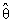= s(X). In the bootstrap world,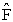generates X* by random sampling giving* = s(X*). There is only one observed value of, but we can generate as many bootstrap replications* as affordable. The crucial step in the bootstrap process is the process by which we construct from x an estimationof the unknown population F. Acha (2014) states that the bootstrap must be applied on the right distribution to get accurate statistical inference. There are many bootstrap methods, but few will be considered in this study, namely the wild and pairwise. According to Davidson and MacKinnon (2000; 2006), When the diagonal hat matrixhave constant variance if the error terms were homoscedastic. There are various ways to specify the distribution of the wild random variable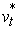with mean 0 and variance 1, but the Mammen distribution is the one that is most commonly used in practice. Apart from that, Davidson and Flachaire (2001) have shown that wild bootstrap tests based on Mammen distribution usually perform better than wild bootstrap from other distributions especially when the conditional distribution of the error terms is approximately symmetric. Many cases, in Davidson and MacKinnon (2008) this procedure seems to be enough for the wild bootstrap DGP to mimic the essential features of the true DGP. As with the residual bootstrap, the null hypothesis can, and should, be imposed whenever we are using the wild bootstrap to test a hypothesis about β. Apart from the fact that wild bootstrap works very well with cross-section data or static models, Gonçalves and Kilian (2004) showed in his work that variants of it can also be used with dynamic models, provided the error terms are uncorrelated. Freedman (1981), proposed the pairwise bootstrap method, the basic idea is to resample the data instead of the residuals unlike the wild bootstrap method. For a detailed discussion of how reliable the bootstrap method is see (Davidson and MacKinnon, 1999). Other authors like Chernick (2007), Lam, and Veall, (2002), Acha (2014), Wolfsegger and Jaki (2006), Wang and Wall (2003), and Zhou (2005) and Davison and Hinkley (1997), William and David (2014), Hall and Wang (2004), Hansen (2000), Lahiri (2005a; 2005b), Lahiri, Lee, and Cressie (2002), Stefan, and Gert (2012), Park and Lee (2001), Robert (1987), Shi, et. al. (2004) have worked extensively on different aspects of bootstrap. However, Lahiri (2003) is the ideal reference for a detailed account of these developments with dependent data and independent data. In addition the smooth bootstrap which is equivalent to sampling from a kernel density estimate of the data is a small amount of (usually normally distributed) zero-centered random noise is added onto each resampled observation while the kernel density is used to we extract all the important features of the data set.

To ascertain the accurate statistical inference and achieve the set objective in this study; the various assessment conditions like the bias, standard error and RMSE will be considered.

### 3. Research Methodology

Secondary data sets are analyzed using bootstrap DGPs and kernel density methods added by the R-statistical package with several assessment conditions as shown under research design.

Consider(3.1)

where the dependent variable, yt is a linear combination of the parameters (but need not be linear in the independent variables), n is the number of observations, β is a k-vector, and the 1 × k vector of regressors X t, which is the tth row of the n × k matrix X, is treated as fixed and µ is an n × 1 vector of independent identically distributed errors with mean 0 and variance σ2. The true distribution of µ is not known.

The corresponding dependent variables from the bootstrap methods are given by;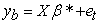(3.2)

For each vector yb the estimator is recomputed and the sampling distribution of the estimator is estimated by the assumed distribution and empirical distribution respectively, of these estimates computed over a large number of yb.

3.1. Research Design

2 Bootstrapping DGPs-: the wild bootstrap DGP is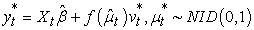(3.3)

where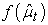is a transformation of the tth residual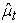, andis a random variable with mean 0 and variance 1. One possible choice foris justbut a better choice is(3.4)

where ht is the tth diagonal of the ‘hat matrix’.

•  A distribution suggested by Mammen (1993)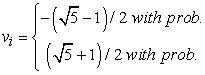(3.5)

The pairs bootstrap DGP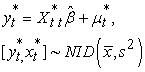(3.6)

•  3 Functional models-: OS3, WB3P and PB3NP

•  3 Ability levels-: N(0,1), N(0,s2), and Ndenoted as (X,Z Q).

•  4 Kernel density approaches-: three test length (shape, center, spread), histogram, density curve, and the quantile-quantile plots (theoretical quantiles and sample quantiles).

•  6 B-levels-: bootstrap levels (19, 99, 199, 499,999, and 1999) were selected using pretest procedure by MacKinnon (2001) and these levels represented typical small, medium, and large bootstrap levels.

•  20 n-levels-: Different sample sizes 10, 14, 20, 28, 40, 50, 56, 80, 113, 160, 200, 226, 320, 452, 500, 640, 905, 1000, 1500 and 2000 were studied. These levels represented typical small, medium, and large sample sizes.

•  Smooth bootstrap-: We want to estimate λn(F) and we can use as an estimate either λn(F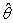) or λn(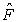n). In fact there is an intermediary choice, that takes the empirical cdfn and smooths it a little, then we use the smoothed empirical cdf denoted byh and we plug it in. This is especially useful when the bootstrap distribution is too discrete, mostly when the statisticis a quantile; the median as we saw in the mouse data analysis had that problem.

•  Kernel density: - Let (x1, x2, …, xn) be an independent and identical distributed sample drawn from some distribution with an unknown density ƒ. We are interested in estimating the shape of this function ƒ. Its kernel density estimator is(3.7)

where K(•) is the kernel — a non-negative function that integrates to one and has mean zero and h > 0 is a smoothing parameter called the bandwidth.

•  Root mean square error of the SEE -: Bias and standard error together define the performance of an estimator, and the root mean square error of the SLR (RMSE) is an index that takes both factors into account.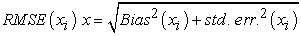(3.8)

Appendix A, the Table 1, Table 2, Table 3 are presented in the order of tests (Bias, Standard error and RMSE).

Appendix B, the Plots (B1-B3 - sample sizes (20, 200, and 2000)) depicting the bootstrapped data sets against the expected values of the corresponding quantiles from the standard normal distribution.

### 4. Analysis of the Dataset

Each of the bootstrap methods were represented by using at least one functional model each from ESS data sets of a particular bootstrap DGP method to illustrate how others were estimated before tabulation;

A. Original Sample (OS3) Model: SLR Equation Estimated from the real data set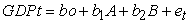(4.1)

OS3 Model, B=99, N(0,1), n=10000: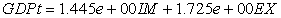(4.2)

Standard error (3.255e-01) (3.420e-02)

Bias (0.0302700) (0.0064000)

RMSE 0.0391

B. Wild Bootstrap DGP (WB3P) Model: SLR Equation Estimated from the wild bootstrap DGP; B=499, N(0.0.9), n=1000; USING (3.3)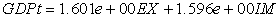(4.3)

Std. Error (2.0010e-01) (3.3148e-01)

Bias (0.15019) (0.0142)

RMSE 0.0500

C. Pairwise bootstrap DGP (PB3P) Model: SLR Equation Estimated from the pairwise bootstrap DGP; B=1999, N(1.0.25), n=200; using (3.6)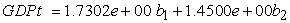(4.4)

Std. Error (1.235e-01) (2.129e-01)

Bias (0.01198) (0.01206)

RMSE 0.0631

Note: the number 3 in the model names represent the three models considered in this study.

### 5. Interpretation of the Result

As described earlier, Root Mean Square Error (RMSE) is an evaluation index with two components: Bias and standard error, expressed as section 3. Results indicate that increasing sample size was associated with smaller RMSE values for all parametric bootstrap models, and the differences among the models also became smaller. When the sample size was 2,000, these differences among the models with higher sample size and bootstrap level were very small. No significant differences were apparent among the three group proficiency levels within each test. However, even when the sample size was 50, the curves of the RMSE yielded by models WB3P and PB3NP were still not stable. It should be pointed out, when the sample size was small, model PB3NP performed worse than models WB3P at most of the estimated points. If the higher bias and standard error was taken into account, it would affect the RMSE significantly. The differences between the models WB3P and PB3NP were less than 0.0006, given that model WB3P, was the best model so far considered in this study based on the fact that it yielded the lowest bias and standard errors.

Interpretation of the real data set in econometric terms using the three models-OS3, WB3P, and PB3NP. The regression coefficients (IM and EX) have positive relationship with GDPtr and also highly significant at 5% level. The 0.9899 and 0.9891 Multiple R-squared and Adjusted R-squared indicate that the model has a good fit of the relationship among the variables and is efficient for prediction. This implies that the model explains about 98% of the import and export of the external sector statistics in Nigeria during the period of 1983-2012. Durbin Watson statistics at 2.842043 suggests that no autocorrelation exist among the variables. The probability value (P-value: < 2.2e-16) indicate that the result is significant and leads to the rejection of the null hypothesis that Nigerian external sector does not contribute significantly to economic growth, thereby accepting the alternative.

### 6. Conclusion

The main findings is that, under all bootstrap conditions, the parametric bootstrap functional models produced smaller bias, standard error and RMSE than the non-parametric bootstrap functional models; when bootstrap level and sample size are large in simple linear regression (SLR). Finally, the kernel density estimates (smooth bootstrap) confirmed that external sector statistics in Nigerian has a chi-square distribution; since the bootstrap distribution created by resampling, also matches the properties of the sampling distribution. An important policy implication is that the distributions of ESS form a good platform for the prediction and forecasting of the Nigeria economy. This is very necessary for the government since the external sector is known to influence important economic indices in Nigeria, as the nation works toward attaining vision 2020 goal of making the economy one of the twenty largest in the world by the year 2020. The limitation that might affect the bootstrap estimates of the bias, standard errors and RMSE may be the ability level differences considered in this study. In that case, it is desirable to investigate if ability level differences might affect the bootstrap estimates of the bias, standard errors and RMSE. This study, therefore, is a stepping stone for further research and prediction especially econometrics and statistics.

### Reference

  Abney, S. (2002). "Bootstrapping", Proceedings of the 40th Annual Meeting of the Association for Computational Linguistics.In article PubMed  Acha, C.K. Bootstrapping Normal and Binomial Distributions,” International journal of econometrics and financial management. 2014, 2 (6).In article  Botev, Z.I.; Grotowski, J.F.; Kroese, D.P. (2010). "Kernel density estimation via diffusion". Annals of Statistics 38 (5): 2916-2957.In article CrossRef  Chernick, M. R. (2007). Bootstrap Methods: A Guide for Practitioners and Researchers, 2nd Edition Wiley, Hoboken.In article CrossRef PubMed  Davidson, R. and Flachaire, E. (2001), The Wild Bootstrap, Tamed at Last, GREQAM Document de Travail 99A32, revised.In article  Davidson, R. and Flachaire, E., (2008). The wild bootstrap, tamed at last, Journal of Econometrics, Elsevier, 146 (1), 162-169.In article CrossRef  Davidson, R & MacKinnon, J. G. (1999).The Size Distortion of Bootstrap Tests, Econometric Theory, Cambridge University Press, vol. 15 (03), pages 361-376, June.In article  Davidson, R. and MacKinnon, J. (2000). Bootstrap tests: how many bootstraps? Econometric Reviews, Taylor and Francis Journals, 19 (1), 55-68.In article  Davidson, R. and MacKinnon, J.G. (2006), ‘Bootstrap Methods in Econometrics’, in Patterson, K. and Mills, T.C. (eds), Palgrave Handbook of Econometrics: Volume 1 Theoretical Econometrics. Palgrave Macmillan, Basingstoke; 812-38.In article  Davison, A. C. and D. V. Hinkley (1997). Bootstrap Methods and Their Application, Cambridge, Cambridge University Press.In article CrossRef  Efron, B., & Tibshirani, R.J. (1993). An introduction to the bootstrap (Monographs on Statistics and Applied Probability 57). New York: Chapman & Hall.In article CrossRef  Freedman, D.A. (1981) Bootstrapping regression models, Ann. Statist. 6, 1218-1228.In article CrossRef  Hall, P., and Wang, Q. (2004). Exact convergence rate and leading term in the Central Limit Theorem for Student’s t-statistic. Ann. Probab. 32, 1419-1437.In article CrossRef  Hansen, B. E. (2000). Testing for structural change in conditional models. J. Econ. 97, 93-115.In article CrossRef  Lahiri, S. N. (2005a). Consistency of the jackknife-after-bootstrap variance estimator for the bootstrap quantiles of a studentizedstatistic. Ann. Statist. 33, 2475-2506.In article CrossRef  Lahiri, S. N. (2003). Resampling Methods for Dependent Data. Springer-Verlag, New York.In article CrossRef  Lahiri, S. N. (2005b). A note on the sub sampling, method under long-range dependence. Preprint, Department of Statistics, Iowa State University.In article  Lahiri, S. N., Lee, Y.-D., and Cressie, N. (2002). Efficiency of least squares estimators of spatial variogramparameters. J. Statist. Plann. Inf. 3, 65-85.In article CrossRef  Lam, J.-P., and Veall, M. R. (2002). Bootstrap prediction intervals for single period regression forecasts. Int. J. Forecast. 18, 125-130.In article CrossRef  Park, E., and Lee, Y. J. (2001). Estimates of standard deviation of Spearman’s rank correlation coefficients with dependent observations. Commun. Statist. Simul. Comput. 30, 129-142.In article CrossRef  Shi, Q., Zhu, Y., and Lu, J. (2004). Bootstrap approach for computing standard error of estimated coefficients in proportional odds model applied to correlated assessments in psychiatric clinical trial. In ASA Proceedings of the Joint Statistical Meetings, pp. 845-854. American Statistical Association, Alexandria, VA. Statist. 8, 296-309.In article  Stefan Van Aelst, GertWillems (2012) Fast and Robust Bootstrap for Multivariate Inference: The R Package FRB 53 (3); 57-62.In article  Wang, F., and Wall, M. M. (2003). Incorporating parameter uncertainty into prediction intervals for spatial data modeled via a parametric variogram. J. Agr. Biol. Environ.In article CrossRef  William G. J. and David A. A. (2014), Bootstrap Confidence Regions for Multidimensional Scaling Solutions. American Journal of Political Science, 58 (1); 264-278. Wolfsegger and Jaki (2006),In article  Zhou, X. H. (2005). Nonparametric confidence intervals for the one-and two-sample problems. Biostatistics, 6, 187-200.In article CrossRef PubMed